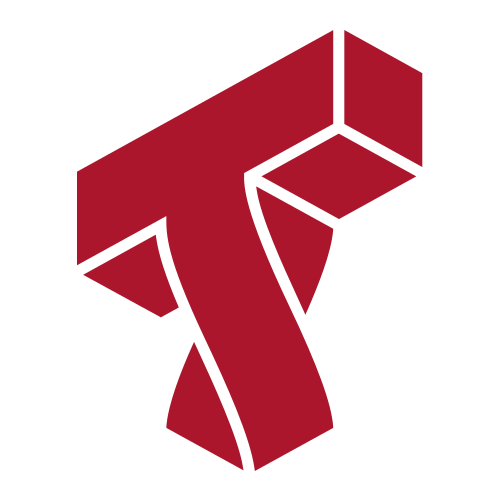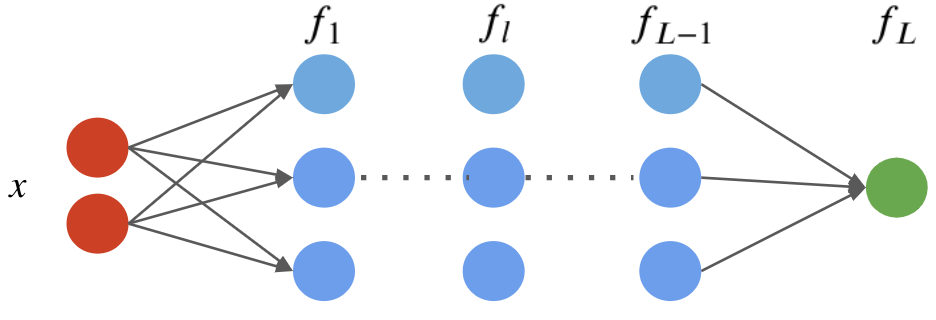# Lecture 15: Deep Learning¶

### Applied Machine Learning¶

Volodymyr Kuleshov, Jin Sun
Cornell Tech

# Part 1: What is Deep Learning?¶

Deep learning is a relatively new and powerful subfield of machine learning closely tied to neural networks.

Let's find out what deep learning is, and then we will see some deep learning algorithms.

# Review: Neural Network Layers¶

A neural network layer is a model $f : \mathbb{R}^d \to \mathbb{R}^p$ that applies $p$ neurons in parallel to an input $x$. $$f(x) = \sigma(W\cdot x) = \begin{bmatrix} \sigma(w_1^\top x) \\ \sigma(w_2^\top x) \\ \vdots \\ \sigma(w_p^\top x) \end{bmatrix},$$ where each $w_k$ is the vector of weights for the $k$-th neuron and $W_{kj} = (w_k)_j$. We refer to $p$ as the size of the layer.

# Review: Neural Networks¶

A neural network is a model $f : \mathbb{R} \to \mathbb{R}$ that consists of a composition of $L$ neural network layers: $$f(x) = f_L \circ f_{L-1} \circ \ldots f_1 (x).$$ The final layer $f_L$ has size one (assuming the neural net has one ouput); intermediary layers $f_l$ can have any number of neurons.

The notation $f \circ g(x)$ denotes the composition $f(g(x))$ of functions

We can visualize this graphically as follows.# What is Deep Learning?¶

In a nutshell, deep learning is a modern evolution of the field of artificial neural networks that emphasizes:

• Very deep neural networks
• Large unstructured datasets, especially images, text, and audio
• Modern computational resources, like GPUs

# Expressivity of Deep Models¶

Why is deep learning powerful? One reason is deep neural networks can represent complex models very compactly.

• Shallow neural networks can represent any function, but need very large hidden layers.
• Deep networks can represent very complex $\mathcal{X} \to \mathcal{Y}$ mappings with fewer parameters.

In practice, deep neural networks can learn very complex mappings such as $\text{image} \to \text{text description}$ that other algorithms cannot.